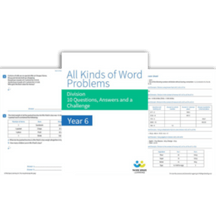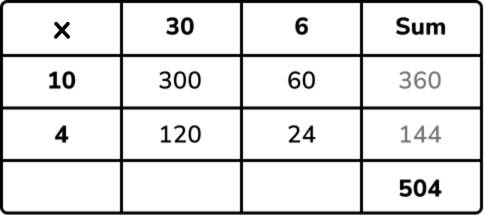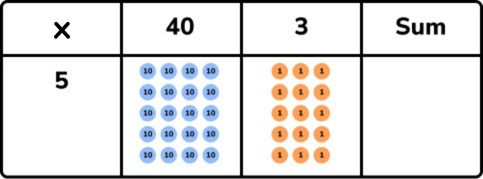Teaching support from the UK’s largest provider of in-school maths tuitionone to one lessonsschools supported

Consolidate learning and plug gaps

In-school online one to one maths tuition developed by maths teachers and pedagogy experts

See it in action

Hundreds of FREE online maths resources!

Daily activities, ready-to-go lesson slides, SATs revision packs, video CPD and more!Explore all resources
Four Operations

Consolidate learning and plug gaps

In-school online one to one maths tuition developed by maths teachers and pedagogy experts

See it in action

# What Is Grid Method Multiplication? Explained For Primary School Teachers, Parents And Pupils

Grid method multiplication is just one method of multiplication used in KS2 primary school maths. In this article, we explain the method and how it compares with other popular methods of multiplication. You will also find a step-by-step explanation of the method, worked examples to consolidate understanding and practice questions to use with your KS2 pupils.

Multiplication is one of the four operations (addition, subtraction, multiplication and division) that are a key part of the maths curriculum throughout primary school. Multiplication is taught right from Year 1, but pupils aren’t required to know more formal written methods of this until Key Stage 2. Grid method multiplication is just one of these written methods.All Kinds Of Word Problems Multiplication

Over 40 multiplication worded problems to extend your pupils’ reasoning skills in multiplication! Includes word problems for Year 3-6Download Free Now!

### What is grid method multiplication?

Grid method multiplication, also known as the box method, is a written method used for multiplication calculations. It can be used from 2-digit by 1-digit multiplication up to 4-digit by 2-digit multiplication (this is all that is required in primary school). The grid method requires the partitioning of numbers, multiplying each place value and adding the constituent parts. See an example below for 324 x 25.

### How does grid method multiplication compare with other popular methods?

There are many other methods of written multiplication – see below how the grid method compares to the lattice method, Chinese stick method and the more commonly seen long multiplication method (expanded and compact).

### Grid method multiplication examples

43 x 5 = 215

#### 4- by 2-digit multiplication

2,647 x 38 = 100,586

2.1 x 3.5 = 7.35

#### Place value counter grid method

Before jumping straight to numbers, you could use place value counters instead.

### How to use grid method multiplication

The grid method of multiplication is a great ‘stepping stone’ to the column method (also known as short or long multiplication) as it clearly lays out each step of the multiplication. Let’s go step-by-step for 36 x 14.

1. Firstly, partition numbers into each place value (in this case, the tens and ones).

2. Write each partitioned part in the top row and first column of the grid. The commutative property of multiplication means either number can be written horizontally or vertically. Pupils will need to have a strong grasp of place value to recognise that, for example, the 3 in the tens column is 30 and the 6 is 6.  (30 and 6 along the top; 10 and 4 down the side).

3. Multiply the tens and units of the first number by the tens and units of the second number and write the answers in the constituent boxes (30 x 10 = 300; 30 x 4 = 120; 6 x 10 = 60; 6 x 4 = 24).

4. Add up all the parts (column addition could be used for this) – this can be done in any order – to find the final answer (300 + 60 = 360, 120 + 24 = 144 and 360 + 144 = 504. In the first example the rows have been totalled, but you could also add the columns first as in the second example – 300 + 120 = 420, 60 + 24 = 84 and 420 + 84 = 504 – to get the same answer).

### When do children learn about grid method multiplication in school?

Children are first introduced to a more ‘formal’ method of multiplication in KS2, starting in Year 3 with multiplying a 2-digit number by a 1-digit number and progressing to larger numbers by multiplying a 4-digit number by a 2-digit number by Year 6. Grid method multiplication isn’t explicitly referred to in the national curriculum – the only formal method referenced in the appendix is the short and long multiplication method.

The national curriculum states that:

• Year 3 pupils should be taught to write and calculate mathematical statements for multiplication and division using the multiplication tables (times tables) that they know, including for two-digit numbers times one-digit numbers, using mental and progressing to formal written methods.
• Year 4 pupils should be taught to multiply two-digit and three-digit numbers by a one-digit number using formal written layout.
• Year 5 pupils should be taught to multiply numbers up to 4 digits by a one- or two-digit number using a formal written method, including long multiplication for two-digit numbers.
• Year 6 pupils should be taught to multiply multi-digit numbers up to 4 digits by a two-digit whole number using the formal written method of long multiplication.

### How does grid method multiplication relate to other areas of maths?

Grid method multiplication itself doesn’t specifically relate to other areas of maths as it is one of several methods used to solve multiplication, however multiplication is required in many other maths topics, such as fractions and area.

### How does grid method multiplication link to real life?

As above, the grid method doesn’t specifically link to real life necessarily, but multiplication itself is used regularly throughout life, such as money (e.g. buying 7 packets of crisps at 45p each) or time (e.g. working out time spent at work if working 8 hours a day, 4 days a week, 37 weeks a year).

### Grid method multiplication worked examples

1) Lauren buys 4 ice creams. Each ice cream costs 85p. How much do they cost altogether?

2) 42.1 x 7

3) In one school year, Chris goes to school for 39 weeks. In each of the weeks, he goes to 34 lessons. How many lessons is that altogether?

### Grid method multiplication practice questions

1. Josh cycles 255 metres in 1 minute. He keeps cycling at the same speed. How far will he cycle in 8 minutes? Answer: 2,040m
2. A factory puts biscuits into packets of 19 biscuits. The factory produces 1,350 packets of biscuits in a day. How many biscuits is that? Answer: 25,650
3. A box of chocolates costs £7. How many do 16 boxes cost? Answer: £112
4. 52.8 x 4 = ? Answer: 211.2
5. Emma buys 5 bunches of flowers. Each bunch of flowers costs £1.20. How much does Emma pay altogether? Answer: £6

### More multiplication resources on our maths hub

Third Space Learning’s maths hub provides a wide range of primary resources for teaching multiplication to Key Stage 2. Our online one-to-one tutoring uses a range of methods, as seen below, to help children to build a strong foundational understanding of key mathematical concepts, such as multiplication.

If you’re looking for more resources around the topic multiplication, take a look at our blog on the best multiplication games to Play at KS1 and KS2 for classroom engagement and our collection of multiplication worksheets that cover all areas of multiplication that students will learn in KS2. Topics include: what is a multiple, factors and multiples, factors, lowest common multiple and highest common factor.

How do you multiply two digit numbers using the grid method?

Partition both numbers, multiply the constituent parts and add them together again.What are the benefits of using the grid method to multiply?

It breaks down the steps of the column method (short and long multiplication) so it can be clearly understood what is happening at each stage.

How do you teach a child multiplication grid method?

Ensure children understand how to partition and represent each place value with counters. Start with 2- by 1-digit multiplication. Show how to multiply each part and add them back together at the end, first with place value counters, then without.Do you have pupils who need extra support in maths?
Every week Third Space Learning’s maths specialist tutors support thousands of pupils across hundreds of schools with weekly online 1-to-1 lessons and maths interventions designed to plug gaps and boost progress.

Since 2013 we’ve helped over 150,000 primary and secondary school pupils become more confident, able mathematicians. Learn more or request a personalised quote for your school to speak to us about your school’s needs and how we can help.

Primary school tuition targeted to the needs of each child and closely following the National Curriculum.##### Sophie Bartlett
Year 6 Teacher
Sophie teaches mixed age classes at a small school in central England. She is a self confessed grammar pedant and number nerd so we've welcomed her as a regular author and editor for Third Space Learning.

Related Articles

x#### All Kinds Of Word Problems Multiplication

Over 40 multiplication worded problems to extend your pupils’ reasoning skills in multiplication! Includes word problems for Year 3-6

#### All Kinds Of Word Problems MultiplicationOver 40 multiplication worded problems to extend your pupils’ reasoning skills in multiplication! Includes word problems for Year 3-6